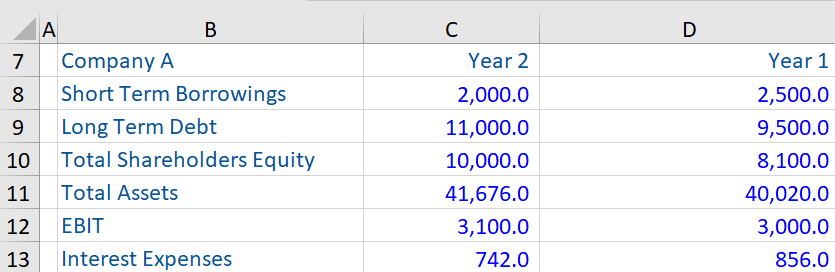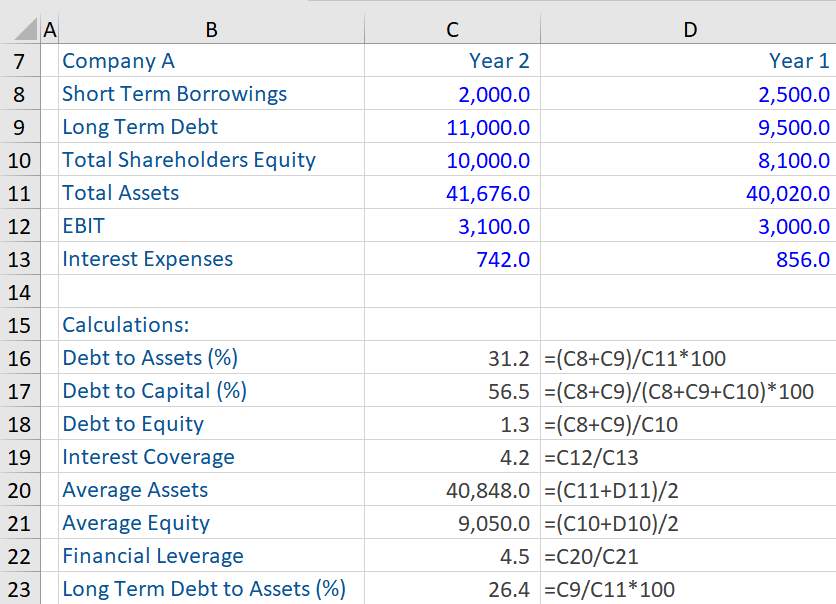## What are “Solvency Ratios?”

The term solvency refers to a company’s ability to pay or meet its long-term financial obligations, which include both interest and principal payments on bank loans or bonds. The ratios that measure this ability are known as “Solvency Ratios.” These ratios assess a company’s long-term financial health and stability and are one of the ways to analyze the balance sheet of a company.

Solvency ratios provide valuable insights into the relative amount of debt that exists in a company’s financial structure and whether it has sufficient cash flows to cover payments related to long-term debt obligations as and when they are due.

## Key Learning Points

• There are six common solvency ratios (among others) used to assess a company’s long-term financial health and stability; and
• The higher (lower) these six ratios are, greater (lower) is the risk of insolvency of a company, with exception of the interest coverage ratio where the higher (lower) the ratio the lower (greater) the risk of insolvency.

## Types of Solvency Ratios

There are six commonly used solvency ratios (among others). These ratios are debt-to-assets, debt-to-capital, debt-to-equity, financial leverage, interest coverage and long-term debt-to-equity. The formulas of these ratios and their interpretation is stated below in brief:

Debt-to-assets ratio = (Outstanding Debt = Short-Term Debt + Long-Term Debt) / Total Assets

This ratio is a measure of financial risk, it measures the extent to which a company’s debt supports its assets. In other words, the percentage of total assets of a company that is funded by debt. The higher this ratio is, the greater the risk of insolvency.

Debt-to-capital ratio = (Outstanding Debt = Short-Term Debt + Long-Term Debt) / (Capital = Short-Term Debt + Long- Term Debt + Total Shareholders’ Equity)

This ratio gives us an idea of a company’s financial structure and measures a company’s total liabilities against its capital. A higher ratio indicates higher risk of insolvency.

Debt to equity ratio = (Outstanding Debt = Short-Term Debt + Long-Term Debt) / Shareholder’s Equity

This ratio measures how much debt a company has relative to equity. A higher (lower) debt-to-equity ratio indicates higher (lower) risk of insolvency.

Financial leverage = Average Assets (Year 1 + Year 2/2) / Average Shareholder’s Equity (Year 1 + year 2/2)

This ratio measures the amount of assets of a company that are supported by each unit (\$) of equity. For example, if this ratio is 3.5, it means that every US\$1 of equity is supporting US\$ 3.5 worth of assets. Therefore, the higher this ratio, the weaker the solvency position of the firm.

Interest coverage = EBIT / Interest Expense

This ratio is a measure of a company’s ability to meet its interest payment on debt obligations with the current earnings before interest and taxes (EBIT). A lower interest rate coverage indicates greater solvency risk and less assurance that a company can service its debt obligations.

Long-term debt to assets ratio = Long Term Debt / Shareholder’s Equity

This ratio is a measure of financial risk, it measures the extent to which a company’s long-term debt supports its assets. In other words, the percentage of total assets of a company that is funded by long-term debt. The higher this ratio, the greater the risk of insolvency.

## Six Solvency Ratios – Example

Given below is the following data (Year 1 and Year 2) from the annual accounts of a company. Based on this information, six solvency ratios (mentioned above) for Year 2 have been computed.The calculation below shows that this company had a debt-to-assets ratio of 31.9% in year 2 i.e., 31.9% of the company’s assets are financed by debt. Likewise, calculations of other ratios can be interpreted per the previously mentioned definitions.